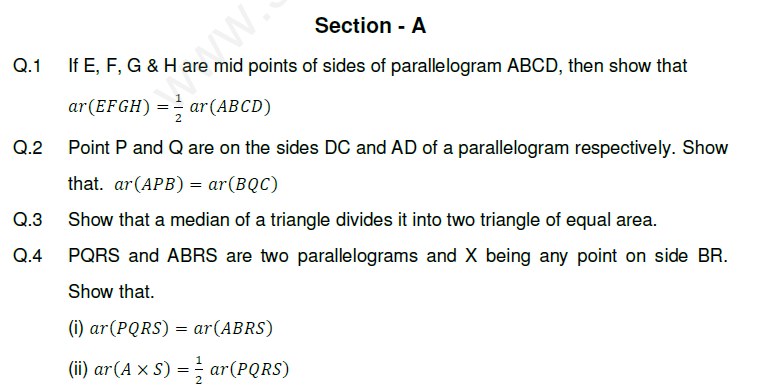# CBSE Class 9 Mathematics Area of Parallelograms and Triangles Assignment Set B

Read and download free pdf of CBSE Class 9 Mathematics Area of Parallelograms and Triangles Assignment Set B. Get printable school Assignments for Class 9 Mathematics. Standard 9 students should practise questions and answers given here for Chapter 9 Areas Of Parallelograms And Triangles Mathematics in Grade 9 which will help them to strengthen their understanding of all important topics. Students should also download free pdf of Printable Worksheets for Class 9 Mathematics prepared as per the latest books and syllabus issued by NCERT, CBSE, KVS and do problems daily to score better marks in tests and examinations

## Assignment for Class 9 Mathematics Chapter 9 Areas Of Parallelograms And Triangles

Class 9 Mathematics students should refer to the following printable assignment in Pdf for Chapter 9 Areas Of Parallelograms And Triangles in standard 9. This test paper with questions and answers for Grade 9 Mathematics will be very useful for exams and help you to score good marks

### Chapter 9 Areas Of Parallelograms And Triangles Class 9 Mathematics Assignment

Concepts and assignment on Area of parallelograms and triangles for CBSE Class IX. Please refer to the attached file for detailed assignment.

Key Concepts

* Area of a parallelogram = (base X height)

* Area of a triangle = ½ X base X height

* Parallelogram on the same base and between the same parallels are equal in area.

* A parallelogram and a rectangle on the same base and between the same parallels are equal in area.

* Triangles on the same base and between the same parallels are equal in area.

* If a triangle and parallelogram are on the same base and between the same parallels, then. (Area of triangle)

* A diagonal of parallelogram divides it into two triangles of equal areas.Click on link below to download CBSE Class 9 Mathematics Area of Parallelograms and Triangles Assignment Set B

 CBSE Class 9 Mathematics Area of Parallelograms and Triangles Assignment Set A CBSE Class 9 Mathematics Area of Parallelograms and Triangles Assignment Set B CBSE Class 9 Mathematics Area of Parallelograms and Triangles Assignment Set C

Tags